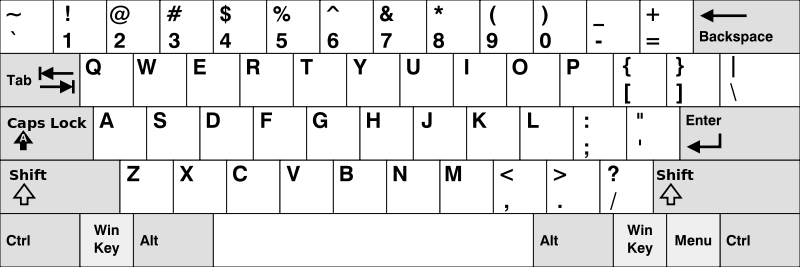500. Keyboard Row

Given an array of strings `words`, return the words that can be typed using letters of the alphabet on only one row of American keyboard like the image below.

In the American keyboard:

• the first row consists of the characters `"qwertyuiop"`,
• the second row consists of the characters `"asdfghjkl"`, and
• the third row consists of the characters `"zxcvbnm"`.Example 1:

```Input: words = ["Hello","Alaska","Dad","Peace"]
```

Example 2:

```Input: words = ["omk"]
Output: []
```

Example 3:

```Input: words = ["adsdf","sfd"]
```

Constraints:

• `1 <= words.length <= 20`
• `1 <= words[i].length <= 100`
• `words[i]` consists of English letters (both lowercase and uppercase).

500. Keyboard Row
``````struct Solution;

use std::collections::HashMap;

impl Solution {
fn find_words(words: Vec<String>) -> Vec<String> {
let rows: Vec<String> = [
"qwertyuiopQWERTYUIOP",
"asdfghjklASDFGHJKL",
"zxcvbnmZXCVBNM",
]
.iter()
.map(|v| (*v).to_string())
.collect();
let mut hm: HashMap<char, usize> = HashMap::new();
for i in 0..3 {
let row = &rows[i];
for c in row.chars() {
hm.insert(c, i);
}
}
let mut res: Vec<String> = vec![];
for word in words {
let rows: Vec<usize> = word.chars().map(|c| *hm.get(&c).unwrap()).collect();
if rows.windows(2).all(|w| w == w) {
res.push(word);
}
}
res
}
}

#[test]
fn test() {